Courses

# The Slope Deflection Method: An Introduction (Part - 1) Civil Engineering (CE) Notes | EduRev

## Civil Engineering (CE) : The Slope Deflection Method: An Introduction (Part - 1) Civil Engineering (CE) Notes | EduRev

The document The Slope Deflection Method: An Introduction (Part - 1) Civil Engineering (CE) Notes | EduRev is a part of the Civil Engineering (CE) Course Structural Analysis.
All you need of Civil Engineering (CE) at this link: Civil Engineering (CE)

Introduction

Introduction As pointed out earlier, there are two distinct methods of analysis for statically indeterminate structures depending on how equations of equilibrium, load displacement and compatibility conditions are satisfied: 1) force method of analysis and (2) displacement method of analysis. In the last module, force method of analysis was discussed. In this module, the displacement method of analysis will be discussed. In the force method of analysis, primary unknowns are forces and compatibility of displacements is written in terms of pre-selected redundant reactions and flexibility coefficients using force displacement relations. Solving these equations, the unknown redundant reactions are evaluated. The remaining reactions are obtained from equations of equilibrium. As the name itself suggests, in the displacement method of analysis, the primary unknowns are displacements. Once the structural model is defined for the problem, the unknowns are automatically chosen unlike the force method. Hence this method is more suitable for computer implementation. In the displacement method of analysis, first equilibrium equations are satisfied. The equilibrium of forces is written by expressing the unknown joint displacements in terms of load by using load displacement relations. These equilibrium equations are solved for unknown joint displacements. In the next step, the unknown reactions are computed from compatibility equations using force displacement relations. In displacement method, three methods which are closely related to each other will be discussed.

1) Slope-Deflection Method
2) Moment Distribution Method
3) Direct Stiffness Method

In this module first two methods are discussed and direct stiffness method is treated in the next module. All displacement methods follow the above general procedure. The Slope-deflection and moment distribution methods were extensively used for many years before the compute era. After the revolution occurred in the field of computing only direct stiffness method is preferred.

Degrees of freedom

In the displacement method of analysis, primary unknowns are joint displacements which are commonly referred to as the degrees of freedom of the structure. It is necessary to consider all the independent degrees of freedom while writing the equilibrium equations.These degrees of freedom are specified at supports, joints and at the free ends. For example, a propped cantilever beam (see Fig.14.01a) under the action of load P will undergo only rotation at B if axial deformation is neglected. In this case kinematic degree of freedom of the beam is only one i.e. θB as shown in the figure.

In Fig.14.01b, we have nodes at A,B,C and D. Under the action of lateral loads p1, p2 and p3, this continuous beam deform as shown in the figure. Here axial deformations are neglected. For this beam we have five degrees of freedom θABC , θD and Δd as indicated in the figure. In Fig.14.02a, a symmetrical plane frame is loaded symmetrically. In this case we have only two degrees of freedom θB and θC. Now consider a frame as shown in Fig.14.02b. It has three degrees of freedom viz. θBC and Δd as shown. Under the action of horizontal and vertical load, the frame will be displaced as shown in the figure. It is observed that nodes at B and C undergo rotation and also get displaced horizontally by an equal amount.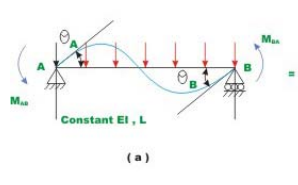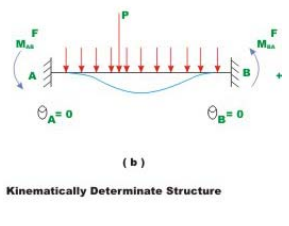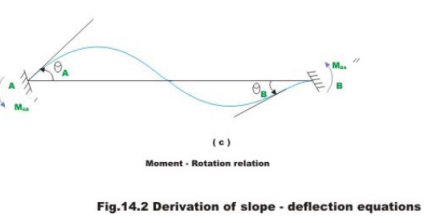Hence in plane structures, each node can have at the most one linear displacement and one rotation. In this module first slope-deflection equations as applied to beams and rigid frames will be discussed.

Instructional Objectives

After reading this chapter the student will be able to

1. Calculate kinematic degrees of freedom of continuous beam.
2. Derive slope-deflection equations for the case beam with unyielding supports.
3. Differentiate between force method and displacement method of analyses.
4. State advantages of displacement method of analysis as compared to force method of analysis.
5. Analyse continuous beam using slope-deflection method.

Introduction

In this lesson the slope-deflection equations are derived for the case of a beam with unyielding supports .In this method, the unknown slopes and deflections at nodes are related to the applied loading on the structure. As introduced earlier, the slope-deflection method can be used to analyze statically determinate and indeterminate beams and frames. In this method it is assumed that all deformations are due to bending only. In other words deformations due to axial forces are neglected. As discussed earlier in the force method of analysis compatibility equations are written in terms of unknown reactions. It must be noted that all the unknown reactions appear in each of the compatibility equations making it difficult to solve resulting equations.
The slope-deflection equations are not that lengthy in comparison. The slope-deflection method was originally developed by Heinrich Manderla and Otto Mohr for computing secondary stresses in trusses. The method as used today was presented by G.A.Maney in 1915 for analyzing rigid jointed structures.

Slope-Deflection Equations

Consider a typical span of a continuous beam AB as shown in Fig.14.1.The beam has constant flexural rigidity EI and is subjected to uniformly distributed loading and concentrated loads as shown in the figure. The beam is kinematically indeterminate to second degree. In this lesson, the slope-deflection equations are derived for the simplest case i.e. for the case of continuous beams with unyielding supports. In the next lesson, the support settlements are included in the slope-deflection equations.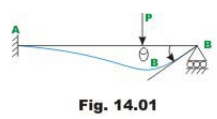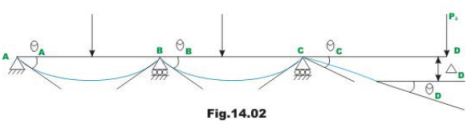For this problem, it is required to derive relation between the joint end moments MAB and MBA in terms of joint rotations θA and θB and loads acting on the beam .Two subscripts are used to denote end moments. For example, end moments Mab denote moment acting at joint A of the member AB. Rotations of the tangent to the elastic curve are denoted by one subscript. Thus, θA denotes the rotation of the tangent to the elastic curve at A. The following sign conventions are used in the slope-deflection equations (1) Moments acting at the ends of the member in counterclockwise direction are taken to be positive. (2) The rotation of the tangent to the elastic curve is taken to be positive when the tangent to the elastic curve has rotated in the counterclockwise direction from its original direction. The slope-deflection equations are derived by superimposing the end moments developed due to (1) applied loads (2) rotation θA (3) rotation θB. This is shown in Fig. 14.2 (a)-(c). In Fig. 14.2(b) a kinematically determinate structure is obtained. This condition is obtained by modifying the support conditions to fixed so that the unknown joint rotations become zero. The structure shown in Fig.14.2 (b) is known as kinematically determinate structure or restrained structure. For this case, the end moments are denoted by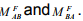The fixed end moments are evaluated by force-method of analysis as discussed in the previous module. For example for fixed- fixed beam subjected to uniformly distributed load, the fixed-end moments are shown in Fig.14.3.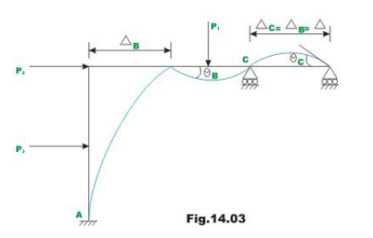The fixed end moments are required for various load cases. For ease of calculations, fixed end forces for various load cases are given at the end of this lesson. In the actual structure end A rotates by θA and end B rotates by θB. Now it is required to derive a relation relating θA and θB with the end moments M'AB and M'BA. Towards this end, now consider a simply supported beam acted by moment M'AB at A as shown in Fig. 14.4. The end moment M’AB deflects the beam as shown in the figure. The rotations θ'A and θ'B are calculated from moment-area theorem.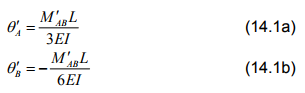Now a similar relation may be derived if only M′BA is acting at end B (see Fig. 14.4).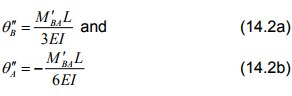Now combining these two relations, we could relate end moments acting at A and B to rotations produced at A and B as (see Fig. 14.2c)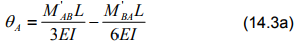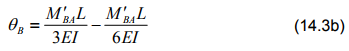Solving for M′AB and M′BA  in terms of θA and θB ,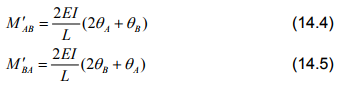Now writing the equilibrium equation for joint moment at A (see Fig. 14.2).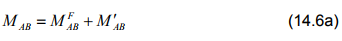Similarly writing equilibrium equation for joint B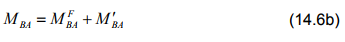Substituting the value of M′AB from equation (14.4) in equation (14.6a) one obtains,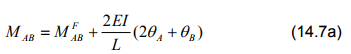Similarly substituting M′BA from equation (14.6b) in equation (14.6b) one obtains,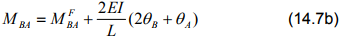Sometimes one end is referred to as near end and the other end as the far end. In that case, the above equation may be stated as the internal moment at the near end of the span is equal to the fixed end moment at the near end due to external loads plus 2EI/L times the sum of twice the slope at the near end and the slope at the far end. The above two equations (14.7a) and (14.7b) simply referred to as slope–deflection equations. The slope-deflection equation is nothing but a load displacement relationship.

Offer running on EduRev: Apply code STAYHOME200 to get INR 200 off on our premium plan EduRev Infinity!

## Structural Analysis

30 videos|122 docs|28 tests

,

,

,

,

,

,

,

,

,

,

,

,

,

,

,

,

,

,

,

,

,

;